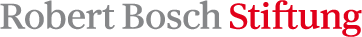# Case-Based Reasoning

Given a large set of problems and their individual solutions case based reasoning seeks to solve a new problem by referring to the solution of that problem which is "most similar" to the new problem. Crucial in case based reasoning is the decision which problem "most closely" matches a given new problem. The basic idea is to define a family of distance functions and to use these distance functions as parameters of local averaging regression estimates of the final result. Then that distance function is chosen for which the resulting estimate is optimal with respect to a certain error measure used in regression estimation. The idea is based on: Dippon J. et al. (2002) .

The R package case-based-reasoning provides an R interface case based reasoning using machine learning.

## Installation

#### CRAN

``````install.packages("CaseBasedReasoning")
``````

#### GITHUB

``````install.packages("devtools")
devtools::install_github("sipemu/case-based-reasoning")
``````

## Features

This R package provides two methods case based reasoning by using an endpoint:

• Linear, logistic, and Cox regression

• Proximity and Depth Measure extracted from a fitted random forest (ranger package)

Besides the functionality of searching similar cases, some additional features are included:

• automatic validation of the key variables between the query and similar cases dataset

• checking proportional hazard assumption for the Cox Model

• C++-functions for distance calculation

## Example: Cox-Beta-Model

### Initialization

In the first example, we use the Cox-Model and the `ovarian` data set from the `survival` package. In the first step we initialize the R6 data object.

``````library(tidyverse)
library(survival)
library(CaseBasedReasoning)
ovarian\$resid.ds <- factor(ovarian\$resid.ds)
ovarian\$rx <- factor(ovarian\$rx)
ovarian\$ecog.ps <- factor(ovarian\$ecog.ps)

# initialize R6 object
coxBeta <- CoxBetaModel\$new(Surv(futime, fustat) ~ age + resid.ds + rx + ecog.ps)
``````

### Similar Cases

After the initialization, we may want to get for each case in the query data the most similar case from the learning data.

You may extract then the similar cases and the verum data and put them together:

Note 1: In the initialization step, we dropped all cases with missing values in the variables of `data` and `endPoint`. So, you need to make sure that NA handling is done by you.

Note 2: The `data.table` returned from `coxBeta\$get_similar_cases` has four additional columns:

1. `caseId`: By this column you may map the similar cases to cases in data, e.g. if you had chosen `k = 3`, then the first three elements in the column `caseId` will be `1` (following three `2` and so on). This means that this three cases are the three most similar cases to case `0` in verum data.
2. `scDist`: The calculated distance
3. `scCaseId`: Grouping number of query with matched data
4. `group`: Grouping matched or query data

### Distance Matrix

Alternatively, you may just be interested in the distance matrix, then you go this way:

`coxBeta\$calc_distance_matrix()` calculates the full distance matrix. This matrix the dimension: cases of data versus cases of query data. If the query dataset is bot available, this functions calculates a n times n distance matrix of all pairs in data. The distance matrix is saved internally in the cbrCoxModel object: `coxBeta\$distMat`.

## Example: RandomForest-Model

### Initialization

In the second example, we present the Random Forest model for a distance measure approximation applied on the `ovarian` data set from the `survival` package. This package offers two ways for distance/similarity calculation (see documentation):

• proximity

• depth

Let's initialize the R6 data object.

All cases with missing values in the learning and end point variables are dropped (`na.omit`) and the reduced data set without missing values is saved internally. You get a text output on how many cases were dropped. `character` variables will be transformed to `factor`.

Optionally, you may want to adjust some parameters in the fitting step of the random forest algorithm. Possible arguments are: , `ntree`, `mtry`, and `splitrule`. The documentation of this parameters can be found in the ranger R-package. Furthermore, you are able to choose the two distance measures:

• `Proximity`: the proximity matrix
• `Depth` (Default): Calculates the average edge length over all trees

This can be done by

All other steps (excluding checking for proportional hazard assumption are the same as for the Cox-Model).

Similar Cases:

Distance Matrix Calculation:

## Contribution

### Responsible for Mathematical Model Development and Programming

• Dr. Peter Fritz

• Professor Dr. Friedel

### FundingThe work was funded by the Robert Bosch Foundation. Special thanks go to Professor Dr. Friedel (Thoraxchirugie - Klinik Schillerhöhe).

# Reference manual

install.packages("CaseBasedReasoning")

0.1 by Dr. Simon Mueller, 2 years ago

Report a bug at https://github.com/sipemu/case-based-reasoning/issues

Browse source code at https://github.com/cran/CaseBasedReasoning

Authors: Dr. Simon Mueller <[email protected]> , PD Dr. Juergen Dippon <[email protected]>

Documentation:   PDF Manual